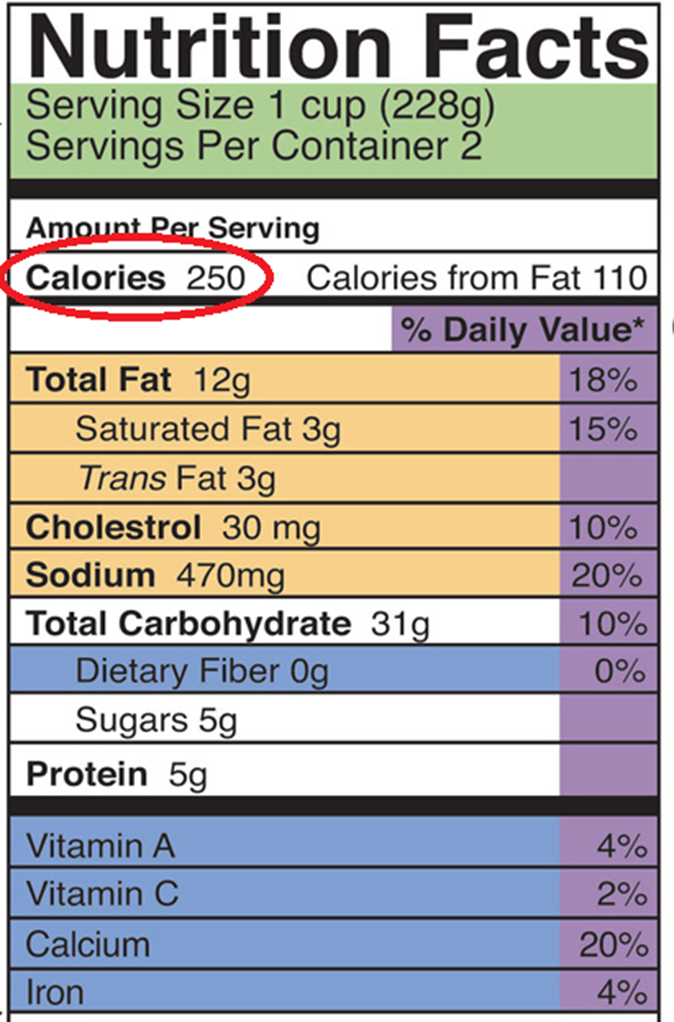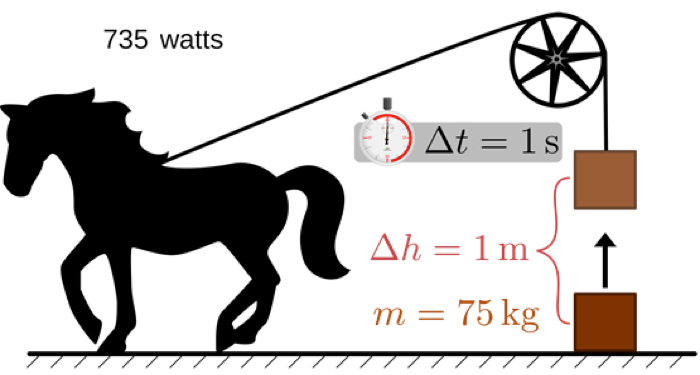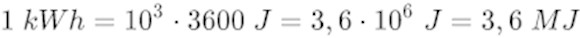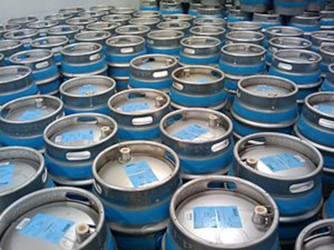Walking, eating, lighting, moving… everything is energy, but why and how to measure these energies… and in which units? Reading texts on energy sources is often made difficult by the multiplicity of units used. It is therefore essential to shed light on this issue.

It is always possible to limit oneself to one unit and its multiples to quantify a physical quantity. For example, time is expressed in the second, the fundamental unit, or one of its multiples such as the hour, the day or the year. This quantification is understood and shared by the whole of humanity.

Nevertheless, many so-called derived units are sometimes substituted for these reference units, which can lead to confusion, difficulties of understanding and conversion problems. For distance, the metre, symbol m, is the international reference in all scientific and technical fields and in the International System of Units (IS) . Nevertheless, the mile, corresponding to 1609m, is widely used in English-speaking countries instead of the kilometre, as are the inch (equivalent to 2.54cm) and the foot (30.48cm).

## 1. The problem of the heterogeneity of energy units

To be able to quantify energy, it is even more nebulous. During the national debate on the energy transition in 2013, members of the public had expressed the request to limit the communication of values to a single unit, which, despite the very legitimate nature of the request, was not granted. The weight of habit in each of the energy domains is one reason for this conservatism, the other being that energy is not perceived as directly as distance or time. It is in fact an abstract concept that applies to different concrete phenomena such as heat, electricity, physical effort. The generic definition of energy is the property of a material system that is conserved during transformations of that system. However, there are many material systems in which this concept of energy is present, and it has taken time to conceive a unity in this diversity. For example, the relationship between the heat given off by a fire and the work used to lift a mass was not elucidated until the 19th century, with thermodynamics (Read: A brief history of energy).

Our multiple units of energy keep track of this history and of the diversity of the material manifestations of energy.

In order to review the main units used for energy, we have to start from what we feel the most in everyday life: heat, food, physical effort. A second important quantity is introduced, power, which expresses the flow of energy, i.e. a variation of energy per unit of time. Examples will then be deduced using the units and providing orders of magnitude for different activities such as food, sports, heating, cars, solar energy, a thermal power plant, all ending with the energy balance of a country.

## 2. The kilocalorie, unit of heatFigure 1. Kilocalories and nutrition facts – source: https://pressbooks.bccampus.ca/sciencehumannutrition/chapter/discovering-nutrition-facts/

All food packaging includes a description of the nutritional value of the product, including, first of all, its energy content expressed in kilocalories, known by the abbreviation kcal (Figure 1).

As with mass , which is expressed in grams, the “kilo” multiple of the calorie unit is usually used because it is the closest to our body’s potential (Figure 2). For example, a morning cereal ration provides about 150 kcal. Our diet should provide an adult with between 1800 and 2600 kcal per day, depending on sex, age, height and physical activity.

The generic definition of a kilocalorie is the amount of heat that raises the temperature of one kilogram of water by one degree Celsius, for example from 14.5 to 15.5°C. According to this definition, a daily food intake of 2100 kcal would raise the average temperature of a 60 kg mass of water by 35°C. This illustrates the fact that the energy provided by the food allows us to maintain body heat, to make the organs work and to fuel intellectual and muscular activity. Secondly, heating water by fire can be done with fuels of varying performance. The combustion of one kilogram of wood, releases about 4300 kcal if it is dry. The combustion of one m3 of gas, which corresponds to a volume of a cube of 1 meter side, brings 9000 kcal and that of one kilogram of domestic fuel 10.000 kcal.

The instrument used to measure these quantities of energy is the calorimeter, a chamber that does not let the heat it contains escape, like the thermos flask for a hot drink (Figure 3).

The fuel burns in the chamber and heats a mass of water in which a thermometer is immersed. The heat energies of combustion can then be measured by knowing the masses of fuel and water involved in the reaction and measuring the temperature rise due to the transformation.

## 3. The kilowatt, the IS unit of power

Consider a physical activity such as cycling or walking. Whether you are accelerating, or climbing a hill, you will soon feel your heart and breathing rates accelerate in response to the effort. Reading a stage report of the Tour de France, we learn that such a rider has reached a power of 300 to 400 watts in the ascent of a hill. For comparison, a bike ride or a mountain hike, at a steady pace, requires about 100 watts.

The definition of a kilowatt, or thousand watts, is the power that moves the point of application of a force of one kilonewton (kN) at a speed of one meter per second, where one kilonewton lifts a mass of 102 kg. Noted with the abbreviation kW, the kilowatt is therefore the value of the power exerted to lift a mass of 102 kilograms one meter per second. This value is reached by a weightlifter during the few seconds of lifting the weight. But this power is physically impossible to maintain in a long-duration endurance sport like long-distance running or cycling.

The unit of power is also used to measure the electrical consumption of household appliances. A hair dryer consumes one kilowatt (1 kW) at full power, an electric heater two to three kilowatts. Other electrical appliances have smaller wattages: an incandescent light bulb consumes 75W and a low-energy light bulb 15W to produce the same amount of light. A television consumes about 250W, as does a laptop. A refrigerator consumes 100W when it is switched on.

## 4. Energy versus power: stock magnitude and flow magnitude

There is a big difference between the two quantities described so far. In the unit of heat, the kilocalorie, there is no reference to time. Heat is a static quantity that reflects the level of a stock. In the experiment to measure the calorific value of a fuel, two states of rest are measured inside the calorimeter: the first before the fuel is ignited, the second long enough after combustion to allow the temperature to become uniform. The heat of combustion is the difference between these two states, regardless of the duration of the transition.

In contrast, the definition of the unit of power contains time. Power is a dynamic quantity and represents a flow. It is a flow of energy that accompanies a transformation of the material system, as for example when lifting a dumbbell. In the same way, a small quantity of powder makes a lot of noise when a firecracker explodes. But the same amount of powder makes no noise when it burns slowly. In the first case, the firecracker explodes because the transformation time is very short and the power is high. In the second case, the powder burns slowly and the power is low. However, the energy of combustion was the same for both experiments.

## 5. The horsepower, another unit of power

This unit of power is not included in the International System (IS) but is still used by engine manufacturers to designate the maximum power of a car, train, plane or boat engine.Figure 4. Horsepower illustration – source : https://www.omnicalculator.com/everyday-life/horsepower

The definition of horsepower is the power developed to lift a 75 kg mass one meter in one second  (Figure 4). This unit, chosen as a reference for steam engines, is of the order of magnitude of the effort that a well-constituted horse can develop. Compared to the power of one kilowatt expended to lift 102 kg at the same speed of one metre per second, the horsepower, lifting a mass of 75 kg, represents a power of 735 W.

Here are some examples of engine power: for an average car, it is 100 hp and six times more for a F1 car. For a high speed train, between 9000 and 12 000 hp depending on the model. But the use of horsepower is gradually disappearing in this sector, leaving the kilowatt as the only power unit. This simplification is a step in the right direction, as long as we keep in mind the order of magnitude that the kilowatt represents, because the horsepower does indeed evoke an order of magnitude.

## 6. The kilojoule, unit of work

Work is a static quantity like heat. However, it differs from heat in that it has a mechanical effect, such as the ascent made by a cyclist. Work is then defined as the product of the action of a mechanical power over a given time. In an equivalent definition, it is the product of the displacement of a force over a given distance.

As with the definition of a kilowatt, a kilojoule is the work done to move a force of one kilonewton over a distance of one metre, for example, to lift a mass of 102 kg one metre. The kilojoule is therefore the energy gain obtained by the action of a power of one kilowatt for one second.

The development of a power of one kilowatt (or, what amounts to the same thing, the lifting of a mass of 102 kg) is beyond human possibilities, except for those of the weightlifter. But the average person can put out a hundred watts of power for ten seconds (or lift 10.2 kg from 10 m), thus doing the same kilojoule of work. Simple mechanical machines, levers or capstans, work on this principle, which makes it possible for human power to accomplish a given task by reducing the power required. On the other hand, the duration of the effort is increased in inverse proportion, because it is necessary in all cases to carry out the work which is the product of the power over the duration.

## 7. The kilowatt-hour, a practical unit of energy

This is another unit of energy constructed from the unit of power, the watt. It is very practical provided that we keep in mind the distinction between power, which is a flow, and energy, which is the variation of a stock by the action of the flow over a given time. The unit of time in the international system being the second, the joule is the energy, or variation of the stock, produced over the duration of one second by a power, or flow, of one watt.

The watt-hour is the same concept extended to a duration of one hour; it is the energy, or variation of the stock, produced during one hour by a power, or flux, of one watt. This unit is more practical because the hour is closer to the duration of everyday life than the second, which is too small. Since the hour has 3,600 seconds, the watt-hour is worth 3,600 joules or 3.6 kilojoules. As for the kilowatt-hour indicated on the electricity or gas meter, it is worth a thousand times more, or 3.6 megajoules.Figure 5. Relation between kWh and J. [Source : https://fr.wikipedia.org/wiki/%C3%89nergie_%C3%A9lectrique]

## 8. The kilojoule, the unit of energy in the international reference system

Until the middle of the 19th century, heat and work were two separate concepts. However, it had been observed that heat could result from the braking of motion, and one theory identified heat as a form of microscopic motion.  Joule, an English physicist, demonstrated this theory experimentally by braking a motion to a near standstill and measuring the heat produced in a calorimeter.

This motion was the rotation of a shaft with blades, all immersed in a highly viscous liquid which brakes the motion.

Let’s assume that the rotor is driven by a cable from which a 102 kg mass is suspended. The descent of this mass by one meter produces a work of one kilojoule. The descent is very slow because of braking; all the work is used to overcome the resistance of the viscous liquid to the movement of the blades. In a calorimeter with a thermal capacity equivalent to one kilogram of water, a temperature rise of 0.24°C is measured, indicating a heat creation of 240 calories.

Joule showed the constancy of this heat creation by destruction of work – 240 calories created for one kilojoule of work destroyed – by multiplying the experiments: various destructions of work in viscous fluids, “braking” of an electric current in a heating resistor. This invariance of the ratio of heat produced by destruction of work is the first principle of thermodynamics. And the joule has become the unit of energy, regardless of its mechanical, electrical or caloric nature.

## 9. Necessary distinction between thermal, mechanical or electrical energy

The joule has thus replaced the calorie in the measurement of energy, whatever its form: mechanical, electrical or caloric. Even in the food industry, where the calorie (or rather the kilocalorie) has remained in use, we see that it is accompanied by its conversion into kilojoules on food labels. This simplification in units may lead to the idea that the caloric and mechanical forms of energy are equivalent. This is not true because Joule’s experiment only concerns the destruction of work that produces heat; only there is all the work converted into heat.

On the other hand, in the reverse transformation, from heat to work, as produced in a heat engine, one can never convert all the heat into work. Only part of the heat can be converted into work and the rest is rejected into the environment. This is the necessary condition for the transformation of heat into work expressed in the second principle of thermodynamics discovered by Carnot (Read: Thermodynamics: the laws, Thermodynamics: energy and entropy and Sadi Carnot face à la fin de la civilisation thermo-industrielle).

In the thermal power plants  that provide much of our electricity, heat is the initial form of energy. It is converted into mechanical action in the turbine, and then electrical action in the alternator. The electrical energy produced is only a part of the thermal energy consumed, according to Carnot’s principle . It is therefore necessary to distinguish between the incoming thermal energy and the electrical energy produced. For this purpose, suffixes are used: kWhth (for thermal) and kWhe (for electrical). The same applies to the power ratings: Wth for thermal power and We for electrical power. Thus a thermal power plant with an electrical production capacity of 1000 MWe consumes a thermal power of 3000 MWth in the boiler. The difference, 2000 MW, is the heat absorbed by the condenser and then rejected into the environment. Some of this heat can then be used for space heating, when the process is profitable.

## 10. The ton of oil equivalent (toe)

This is the unit currently used in economic studies and for the annual energy balances of the various countries in the European Union (EU) and the world. A few decades ago, when mineral coal was the main source of energy consumed in the world, the reference was the tonne of coal equivalent (toe). The toe measures the heat produced by the combustion of one tonne of oil . The standard value is 41.9 GJ (gigajoules) or 11.6 MWh (megawatt hours).Figure 6. Oil as a reference for energy combustion counting – source : https://en.wikipedia.org/wiki/Barrel_(unit)#Oil_barrel

The use of toe in energy balances complicates rather than simplifies the presentation of energy issues. Indeed, the same balances use the MWh to quantify electricity production and the GJ to quantify heat production. But how can we appreciate the orders of magnitude and the relationships between the different forms of energy when their values are expressed in several units? As difficult as when the prices of products are expressed in different currencies. The toe can hardly play this role of universal unit because it will not withstand the end of oil’s domination. Is it not paradoxical to talk about the imminent end of oil while continuing to use toe in national or international energy balances? Not to mention the curiosity of measuring wind production in toe.

## Conclusion: for a more comprehensible unit in annual balances

Every subscriber to the electricity network receives consumption statements in kWh. It is the same for gas or heat. In the interests of public understanding, it would therefore be preferable to use the kWh or its multiples to unify the balances for all energy sources. This would already be a great step forward.

Annual balances could also be established in kilowattyear (kWy). The definition is simple: while the kWh is the quantity of energy consumed to deliver a power of one kW for one hour, the kWy is the quantity of energy consumed to deliver this same power for a year of 365 days, i.e. 8,760 hours. One kWy is therefore worth 8760 kWh.

The energy balances of countries, sub-continents or continents, such as the European Union, Africa, or the world are always given for one year and include all energy sources. The clearest and simplest way would be to express them in a unit that is :

• independent of any particular energy (such as kWh or kWy)
• consistent with the duration of the measurement, the year, which is the case for kWy.

The reference to the year to measure energy in this context of energy balances has the advantage of providing an immediate comparison between energy production and the installed production capacity of hydroelectric, conventional thermal or nuclear power plants as well as solar or wind farms.It should not be confused with the fiscal horsepower (HP) in the case of cars, which depends on the standardised value of carbon dioxide (CO2) emissions in grams per kilometer (g/km) and the maximum power of the engine (in kilowatts or horsepower).

## Notes and references

 The name International System of Units, also known by the abbreviation IS, was officially adopted by the General Conference on Weights and Measures at its 11th meeting in 1960. It consists of a set of seven base units: the metre (distance), the kilogram (mass), the second (time), the ampere (electric current), the kelvin (temperature), the mole (amount of matter) and the candela (luminous intensity). In addition, there are prefixes and derived units. See Bureau International des Poids et Mesures.

 The mass is a fundamental physical property of a body and reflects the amount of matter contained in it. See Futura Sciences.

 It should not be confused with the fiscal horsepower (HP) in the case of cars, which depends on the standardised value of carbon dioxide (CO2) emissions in grams per kilometer (g/km) and the maximum power of the engine (in kilowatts or horsepower).

 Or the theory of heat transfer which corresponds to a microscopic energy transfer producing heat

 James Joule (1818-1889) studied the nature of heat and discovered its relationship to mechanical work, which led to the theory of conservation of energy, the first law of thermodynamics. He also formulated a relationship between the electric current flowing through a resistor and the heat dissipated by it, known in the 20th century as Joule’s law U=RI. Finally he worked with Lord Kelvin to develop the absolute temperature scale and studied magnetostriction. In the International System, the unit of energy and heat quantity bears his name: the joule.

 Thermal power plants are all based on a heat source that feeds a thermodynamic cycle: heat from the combustion of gas, fuel oil or biomass in conventional power plants, heat from the fission of uranium in nuclear power plants, concentrated solar heat in thermodynamic solar power plants or heat from the earth’s crust in geothermal power plants.

 The French scientist Sidi Carnot published a scientific work on thermal machines in 1824 and demonstrated that it is impossible to achieve a maximum efficiency of 100% for thermal machines.

 This measure has been standardized because there are variations in the energy content of the resource depending on the origin of the oil.

The Encyclopedia of Energy is published by the Association des Encyclopédies de l’Environnement et de l’Énergie(www.a3e.fr), contractually linked to Grenoble Alpes University and Grenoble INP, and sponsored by the Academy of Sciences.

To cite this article, please mention the author’s name, the title of the article and its URL on the Encyclopedia of Energy website.

The articles of the Encyclopedia of Energy are made available under the terms of the license Creative Commons Attribution – Noncommercial – No Derivative Works 4.0 International.